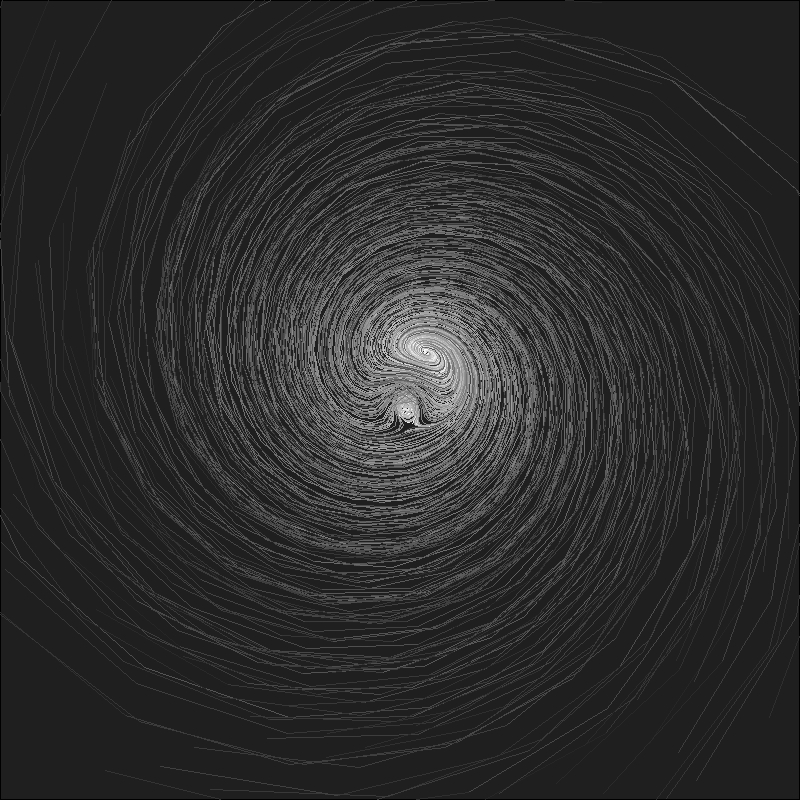# The Ikeda’s Galaxy

Chaos is the score upon which reality is written (Henry Miller)

Nonlinear dynamical systems are an enormous seam of amazing images. The Ikeda Map is an example of strange attractor which represents the movement of particles under the rules of certain differential equations.

I have drawn the trajectories followed by of 200 particles under the 2D-Ikeda Map with the same tecnique I used in this previous post, resulting this nice galaxy:Wold you like to create your own galaxies? Here you have the code:

```u=0.918 #Parameter between 0 and 1
n=200 #Number of particles
m=40 #Number of iterations
ikeda=data.frame(it=1,x1=runif(n, min = -40, max = 40), y1=runif(n, min = -40, max = 40))
ikeda\$x2=1+u*(ikeda\$x1*cos(0.4-6/(1+ikeda\$x1^2+ikeda\$y1^2))-ikeda\$y1*sin(0.4-6/(1+ikeda\$x1^2+ikeda\$y1^2)))
ikeda\$y2=  u*(ikeda\$x1*sin(0.4-6/(1+ikeda\$x1^2+ikeda\$y1^2))+ikeda\$y1*cos(0.4-6/(1+ikeda\$x1^2+ikeda\$y1^2)))
for (k in 1:m)
{
df=as.data.frame(cbind(rep(k+1,n),
ikeda[ikeda\$it==k,]\$x2,
ikeda[ikeda\$it==k,]\$y2,
1+u*(ikeda[ikeda\$it==k,]\$x2*cos(0.4-6/(1+ikeda[ikeda\$it==k,]\$x2^2+ikeda[ikeda\$it==k,]\$y2^2))-ikeda[ikeda\$it==k,]\$y2*sin(0.4-6/(1+ikeda[ikeda\$it==k,]\$x2^2+ikeda[ikeda\$it==k,]\$y2^2))),
u*(ikeda[ikeda\$it==k,]\$x2*sin(0.4-6/(1+ikeda[ikeda\$it==k,]\$x2^2+ikeda[ikeda\$it==k,]\$y2^2))+ikeda[ikeda\$it==k,]\$y2*cos(0.4-6/(1+ikeda[ikeda\$it==k,]\$x2^2+ikeda[ikeda\$it==k,]\$y2^2)))))
names(df)=names(ikeda)
ikeda=rbind(df, ikeda)
}
plot.new()
par(mai = rep(0, 4), bg = "gray12")
plot(c(0,0),type="n", xlim=c(-35, 35), ylim=c(-35,35))
apply(ikeda, 1, function(x) lines(x=c(x,x), y=c(x,x), col = paste("gray", as.character(min(round(jitter(x*80/(m-1)+(20*m-100)/(m-1), amount=5)), 100)), sep = ""), lwd=0.1))
```